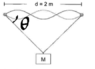# Tension in a String: Solve for Unknown Lengths - 98.6N

• snormanlol

#### snormanlol

Homework Statement
A mass M (15kg) is hanging on a string which is forming a closed loop (total length of the loop = 5m, with mass per unit length=2*10^(-3) kg/m). The string runs trough 2 mass and frictionless wheels, with a distance of 2 meters between them. Determine the tension T in the string.
Relevant Equations
F = m*a;
τ=I*α
I know that the answer has to be 98.6N. So I know that Fy=0 so that 2*T*sin(theta) = 147.25. Then I was think to take the torque of the left wheel but I can't find the lever arm of the tension force. I also know that u can solve the question by saying that the 2 sides of unknown length are 1.5 m but I'm not sure why u can do that.
Here is a picture of the problem:

#### Attachments

•1566828920571.png
5.7 KB · Views: 107
well I think this equation ##2T\sin\theta=147.25## is correct. All you need is a geometric argument to determine ##\theta##. From the figure it seems that we can take the triangle to be isosceles. So how can you find ##\theta## if you know that the triangle is isosceles, has perimeter 5m and base 2m?

•snormanlol
Well we can take Arctan(1/1.5) but I don't see why we can say that the perimeter is 5. Isn't the loop 5 m? And the length of the sides are unknown? That's why I tried using torque but I got the equation 2*T*sin(theta)=147.25 back.

What do you mean , since the total loop length is 5m, can't we say that the perimeter of the triangle is 5m?
Btw that should be ##\theta=\arccos(1/1.5)##..

•SammyS
Isn't the loop 5 m?
Maybe the diagram, with its waveform in the horizontal section, is misleading you. Ignore the waveform at this stage (that is presumably for a later part of the question) and treat the horizontal part as straight. So the loop of string forms a triangle, and the length of the loop is the perimeter.

Ah yes it misleaded me thank you guys and yeah I meant arccos but I made a mistake. But thank you for the help guys.

•Delta2